# Algebra II : Complex Imaginary Numbers

## Example Questions

← Previous 1 3 4 5 6 7

### Example Question #2 : Irrational Numbers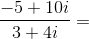Possible Answers: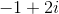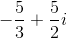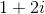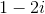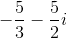Correct answer:Explanation: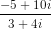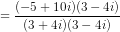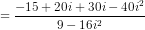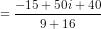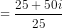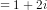### Example Question #3 : Irrational Numbers

Multiply: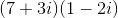Possible Answers: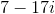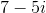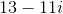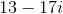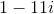Correct answer:Explanation:

Use the FOIL technique: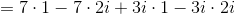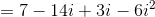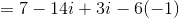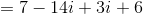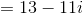### Example Question #5 : Irrational Numbers

Evaluate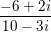Possible Answers: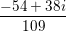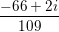You cannot divide by complex numbers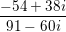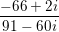Correct answer:Explanation:

To divide by a complex number, we must transform the expression by multiplying it by the complex conjugate of the denominator over itself. In the problem,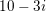is our denominator, so we will multiply the expression by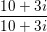to obtain: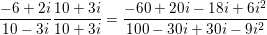.

We can then combine like terms and rewrite all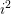terms as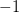. Therefore, the expression becomes: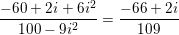Our final answer is therefore### Example Question #6 : Irrational Numbers

Simplify the following product: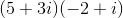Possible Answers: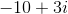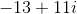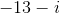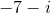Correct answer:Explanation:

Multiply these complex numbers out in the typical way: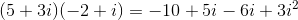and recall that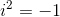by definition. Then, grouping like terms we get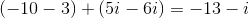which is our final answer.

### Example Question #7 : Irrational Numbers

Identify the real part of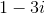Possible Answers:

none of the above.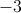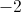Correct answer:Explanation:

A complex number in its standard form is of the form: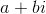, wherestands for the real part andstands for the imaginary part. The symbolstands for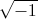.

The real part in this problem is 1.

### Example Question #8 : Irrational Numbers

Simplify: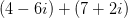Possible Answers: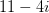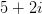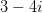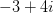Correct answer:Explanation:

To add complex numbers, find the sum of the real terms, then find the sum of the imaginary terms.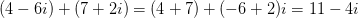### Example Question #1 : Complex Imaginary Numbers

Simplify: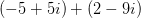Possible Answers: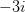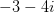Correct answer:Explanation:

To add complex numbers, find the sum of the real terms, then find the sum of the imaginary terms.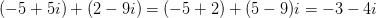### Example Question #2 : Imaginary Numbers

Simplify: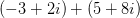Possible Answers: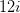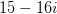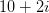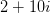Correct answer:Explanation:

To add complex numbers, find the sum of the real terms, then find the sum of the imaginary terms.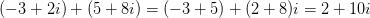### Example Question #3 : Imaginary Numbers

Simplify: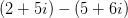Possible Answers: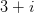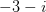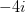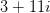Correct answer:Explanation:

To subtract complex numbers, subtract the real terms together, then subtract the imaginary terms.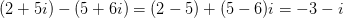### Example Question #4 : Imaginary Numbers

Simplify: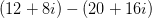Possible Answers: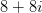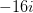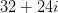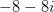Correct answer:Explanation:

To subtract complex numbers, subtract the real terms, then subtract the imaginary terms.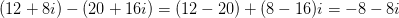← Previous 1 3 4 5 6 7

### All Algebra II Resources